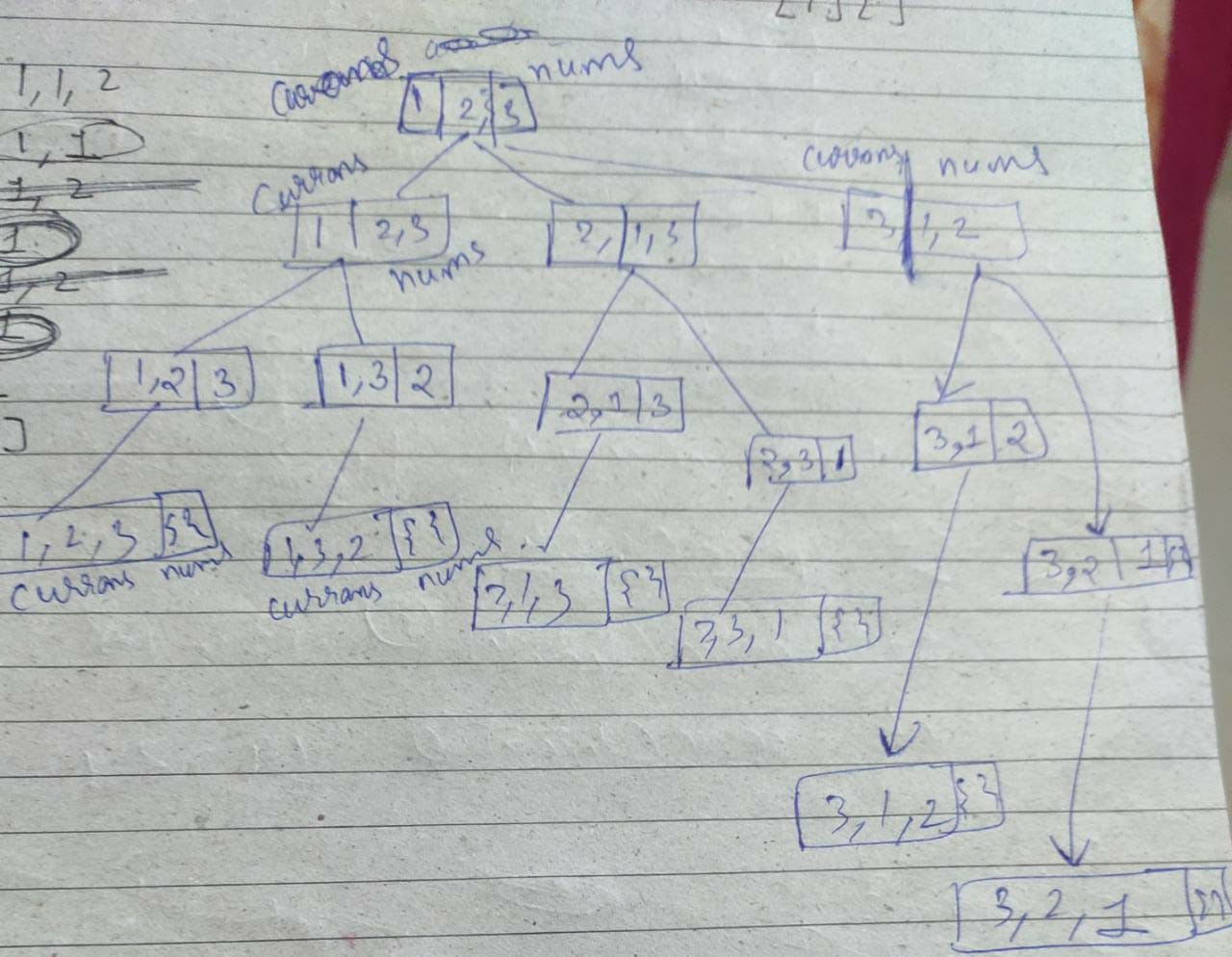# QUESTION 1 — Permutations

Given a collection of distinct integers, return all possible permutations.

Example:

`Input: [1,2,3]Output:[  [1,2,3],  [1,3,2],  [2,1,3],  [2,3,1],  [3,1,2],  [3,2,1]]`

I will discuss 2 different thought processes and solutions for this question. Both approaches involve Backtracking.

## THOUGHT PROCESS FOR BACKTRACKING SOLUTION — 1

Let's revise the backtracking Brahmastr before moving on, as we will follow it anyways in the approach.

# The Backtracking Brahmastra :

1. Find what choice(s) we have at each step. What different options are there for the next step?
2. For each valid choice:

(a)Make it and explore recursively. Pass the information for a choice to the next recursive call(s).

(b)Undo it after exploring. Restore everything to the way it was before making this choice.

3. Find our base case(s). What should we do when we are out of decisions?

Let’s fit in our question :

1. Find what choice(s) we have at each step.

In the currans to include any of the n available numbers. Let’s take an example and try to understand, What I mean by saying “having the choice to include any of the n available numbers”. Let’s say input = [1,2,3], currently the currans is {}, Choice to add to currans — 1,2, or 3,

2. For each valid choice:

(a)Make it and explore recursively. Pass the information for a choice to the next recursive call(s).

Make the choice of including 1, 2 or 3 in the currans, with this line `currans.push_back(nums[i])`

Explore recursively `helper(nums,currans)` i.,e explore all the possible answers starting with ith choice, let's say i = 0, nums[i] = 1, so this call is meant to explore all the permutations starting with 1, so when you return from this call, you can expect that recursion would have added [1,2,3], [1,3,2] in your final answer.

(b)Undo it after exploring. Restore everything to the way it was before making this choice.

Now it’s time to revoke the previous choice and restore things to as they were before making this particular choice. Hence, `currans.pop_back()`

3. Find our base case(s). This one should be an easy guess if the currans’ size is equal to input’s size, we can conclude we made a permutation and push it back into our answer and return from the call.Typical decision tree (pardon for the poor handwriting)
`class Solution {public:    vector<vector<int>> ans;        void helper(vector<int>& nums, vector<int>& currans){        if(currans.size() == nums.size()){            ans.push_back(currans);            return;        }               for(int i = 0; i < nums.size(); i++){ //if the currans is already having nums[i], we skip this call(won't //work in case of duplicates)          if(find(currans.begin(),currans.end(),nums[i]) !=       currans.end()) continue;            currans.push_back(nums[i]);            helper(nums,currans);            currans.pop_back();        }            }    vector<vector<int>> permute(vector<int>& nums) {            vector<int> currans = {};            helper(nums,currans);            return ans;    }    };`

## THOUGHT PROCESS FOR BACKTRACKING SOLUTION — 2

The idea is to swap each of the remaining characters in the string with its first character and then find all the permutations of the remaining characters using a recursive call. The base case of the recursion is when the string is left with only one unprocessed element. Below is the recursion tree for printing all permutations of string “ABC”

`#include <bits/stdc++.h>using namespace std;void permute(string s, int i, int n){    if(i == n){        cout << s << " ";           return;      }for(int j = i; j < n; j++){      swap(s[j],s[i]);       permute(s,i+1,n);      swap(s[j],s[i]);  }}int main(){    string s;    cin >> s;    permute(s,0,s.size()-1);}`

# QUESTION 2 — Permutations II

Given a collection of numbers that might contain duplicates, return all possible unique permutations.

Example:

`Input: [1,1,2]Output:[  [1,1,2],  [1,2,1],  [2,1,1]]`

The core idea of the solution is gonna be the same as the 1st solution that we discussed above. We just need to take care that we don’t make any duplicate recursive calls, for which we use a boolean array used(I know I have used an integer vector) if the ith number has already been pushed into currans i.e, if `used[i] = 1` or if `nums[i] == nums[i-1] and nums[i-1] was already used to make the call, we won't make another call with the same params`(obviously we have sorted the array beforehand to make sure duplicate elements occur together and we can recognize and skip duplicate calls)

`class Solution{public:  vector<vector<int>> ans;void helper(vector<int> &nums, vector<int> &currans, vector<int> &used)  {    if (currans.size() == nums.size())    {      ans.push_back(currans);      return;    }for (int i = 0; i < nums.size(); i++) {  if(used[i] == 1 || i > 0 && nums[i]==nums[i - 1] && used[i-1]==1)        continue;      used[i] = 1;      currans.push_back(nums[i]);      helper(nums, currans, used);      used[i] = 0;      currans.pop_back();    }  }vector<vector<int>> permuteUnique(vector<int> &nums)  {    vector<int> currans = {};    vector<int> used(nums.size(), 0);    sort(nums.begin(), nums.end());    helper(nums, currans, used);    return ans;  }};`

PS: All the problem statements are taken from leetcode.com

--

--